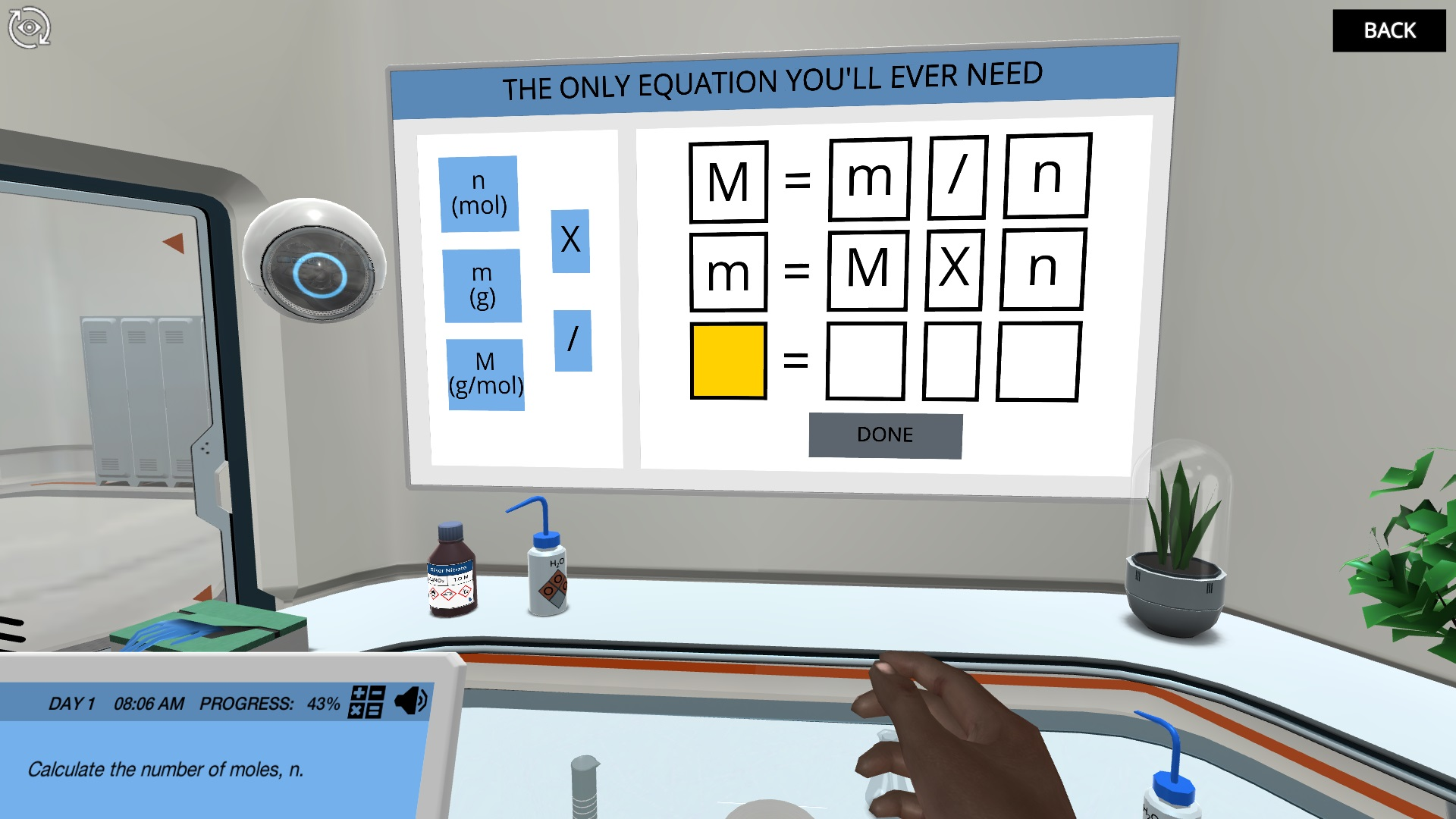Stoichiometric Calculations: Identify a compound using gravimetric analysis

Learn about Avogadro’s number and the relationship between moles, mass and molecular weight as you use the technique of gravimetric analysis to identify an unknown compound.Try our lab safety simulation

Discover one of our 200+ learning simulations today

• Description
• Features

Did you know that there are more water molecules in a glass of water than there are sand grains in the Sahara desert? In this simulation you will learn about the relationship between mass, molecular weight and the number of atoms or molecules and you will understand the magnitude and importance of Avogadro’s number.

Identify an unknown compound

In order to identify a compound where the label has been partly destroyed, you must apply the technique of gravimetric analysis. To do so, you must first learn to understand the relationship between mass, moles and molecular weights and how to perform stoichiometric calculations from mass to mass via conversions to mole.

Stoichiometric calculations with moles

You will perform a realistic gravimetric analysis with detailed instructions on what to do and why to do it in every step of the experiment. From balancing the equation to recognizing the stoichiometry of the reactants and finding out which equation to employ in the calculations, the theory behind the experiment is explained step-by-step in the order of the experiment.

What compound is it?

At the end of the simulation, you will have finalized all of the stoichiometric calculations and the answer to the question should be clear… Can you see what compound it is?

Get started now

Learn about Avogadro’s number and the relationship between moles, mass and molecular weight as you use the technique of gravimetric analysis to identify an unknown compound.

Techniques in lab
Gravimetric analysis
Learning objectives

At the end of this simulation, you will be able to...

• Explain the relationship between mass, molecular weight, and numbers of atoms or molecules and perform calculations deriving these quantities from one another
• Perform mass-to-mass stoichiometric calculations via conversions to mole
• Identify the limiting and excess reagents in a chemical reaction
• Calculate the theoretical, actual and percent reaction yield
• Define Avogadro’s number and describe the mole quantification of matter
Simulation features

Length: 35
Accessibility mode: Available
Languages: English (United States)

NGSS

HS-PS1-1, HS-PS1-2

IB

1.3 Reacting masses and volumes

1.2 The mole concept

1.1 Introduction to the particulate nature of matter and chemical change

AP

1.3 Elemental Composition of Pure Substances

4.5 Stoichiometry

4.3 Representation of Reactions

1.1 Moles and Molar Mass

4.2 Net Ionic Equations

4.1 Introduction for Reactions